## Confidence Interval for Population Mean, μ, based on the sample mean when σ is known

There is a great deal of material to cover before we really get to confidence intervals. Fortunately, we have done that work in previous pages. We summarize that work in Table 1.

 Table 1 Probability We have discrete and continuous probability distributions. For continuous probability distributions we can show a curve of the probability density function. The area under the entire curve is 1.00. The area under the curve and to the left of a value v is the probability that the random variable X is less than v. This is written as P(X -v). For two values a and b, where a < b, we have P( a < X < b) = 1 - P(Xb). The Normal Distribution The normal distribution is a continuous probability distribution. The normal distribution is based on a mathematical formula. The standard normal distribution has mean=0 (μ=0) and standard deviation=1 (σ=1). We have a table to find the area under the standard normal distribution probability density function curve and to the left of a value z, i.e., P(X

With all of this, consider the following situation. First, let us find the z-score in N(0,1) such that P(X<z)=0.025. We will do this with qnorm(0.025) as shown in Figure 1;

Figure 1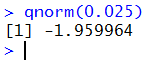That value is so close to -1.96 that we will use the rounded value for the rest of this discussion. The meaning of the -1.96 is that for a N(0,1), 2.5% of the area under the curve is to the left of -1.96. Since the normal distribution is symmetric, this means that P(X>1.96)=0.025 also. Thus, 95% of the area is between -1.96 and 1.96. Remember that in our standard normal distribution the standard deviation is 1. Therefore, we really could have said that 95% of the area is within 1.96 standard deviations from the mean. This will be true of any normal distribution.

Now, we start with a population that has a known standard deviation, σ. It also has a known mean, μ. We plan to take a sample of size 36. We know that the distribution of sample means from size 36 samples is N(μ,σ/sqrt(36)), or simplifying, N(μ,σ/6). If that is the distribution of the means of size 36 samples, then we know that 95% of the samples that we take will have a sample mean that is between μ-1.96*σ/6 and μ+1.96*σ/6. This is shown at the top of Figure 2 where the point a is 1.96 standard deviations below the mean and point b is 1.96 standard deviations above the mean. Again, given the distribution of the sample means, if we take repeated samples of size 36, then 95% of those samples will have a sample mean that falls between a and b.

Figure 2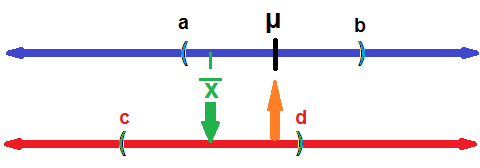We take our sample. It turns out that our sample mean is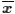and, for this part of the discussion, we will assume that it is one of the 95% of the sample means that falls between a and b. We see this in Figure 2. Then, on a new line, the lower one in Figure 2, let us look at the interval that is just as wide as was the one from a to b. In Figure 2 that interval is from c to d.

Then, it must be true, ifis in the interval (a,b), then the mean, μ, must be in the interval (c,d). Try it again. Choose any point in (a,b), drop down to the lower line, then construct a new interval around that value on the lower line (using the same width interval). The value of the true mean, μ, will have to be in that new interval.

Similarly, if you choose to putoutside of the interval from a to b (so it is one of the 5% of the sample means that fall outside of the interval), then it must be the case that the true mean, μ is not in that new interval.

This is a spectacular result! It tells us that if we take a sample of size 36 from a population with a known standard deviation, let us say it is 12, and if we find that the sample mean turns out to be 43, then we can construct an interval around that value of 43 and make that interval go from 43-1.96*12/6 = 43-1.96*2 = 43-3.92 = 39.08 to 43+1.96*12/6 = 43+1.96*2 = 43+3.92 = 46.92. We have no way of knowing if the true mean of the population is in that interval, but we can say that if we follow this same procedure over and over, 95% of the intervals that we would construct will contain the true mean. This is a 95% confidence interval.

The beauty of this is that we did not need to know the population mean to do this. In fact, if we know the population mean then there is no sense in finding a confidence interval for the population mean. This procedure is to be used when we do not know the population mean but we want to make a good guess as to the value of that mean.

Our best point estimate for the population mean is the sample mean. However, the point estimate is almost certainly wrong. It might be off by a little; it might be off by a lot, but it is almost always off. What we want is an interval of values where we can be relatively certain that our method of creating the interval tells us that some specified high percentage of the intervals created this way do in fact hold the population mean. The little twist to this is that to use our method we need to know the population standard deviation.

If we know σ, for a new case we say it is 3.41, then we can choose a level of significance, say 90%. We calculate the percent we are missing, 100%-90%=10%. We figure that we need half of that 10% below and half of that above our interval. In this case we would have 5% below and 5% above. Since sample means form a normal distribution, we can find the z-score that has 5% to its left by using qnorm(0.05). This turns out to be approximately -1.645. We set the size of the sample; in this case we will use a sample of size 30. With the population standard deviation being 3.41, the standard deviation of the means of samples of size 30 will be 3.41/sqrt(30)≈3.41/5.4772≈0.62258. We take the sample and find that=94.2. Then we construct our 90% confidence interval by taking±-1.645*3.41/sqrt(30) or 94.2±(-1.645*0.62258) or 94.2±-1.02414 or from 93.175859 to 95.22414, which we might express in rounded form as (93.176,95.224). These computations are shown in Figure 3.

Figure 3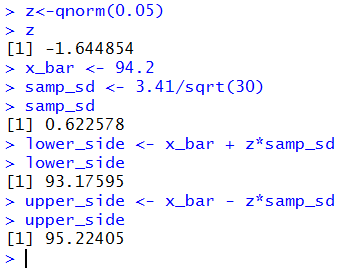A few notes on this before we state the general formula, look at numerous examples, and then look at automating the process. First, to do this we need to know four things: the population standard deviation, the sample size, the desired confidence interval, and finally the sample mean. Second, by looking at the left tail, asking as we did above for the z-score such that P(X<z)=0.05, the result stored in x will be a negative value. That is why we use addition in `lower_side <- x_bar + z*samp_s`. There are many ways for us to have changed the value in z to be the positive side of the pair, and then we would have been able to use a more sensible subtraction to get the lower_side and an addition to get the upper_side. Third, and I wanted to sneak this in where few people will read it, this type of problem is at best an academic exercise. We are supposed to know the population standard deviation without knowing the population mean. To find the standard deviation we need to know either the mean for one formula or the sum of the values and the number of values for the other. Still, we need to do this because it sets the stage for a more realistic problem, finding the confidence intervals when we know neither the mean nor the standard deviation of the population

### The General Formula

In general, we start with a population, and we know that the population standard deviation is σ. We determine a confidence level, cl. We determine a value we will call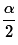read as "alpha over 2", which is half the area not in the confidence interval. Thus,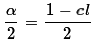. Usingwe find the z-score such that P(X < z)=. We refer to that z-score as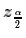. We determine a sample size n and take a sample of size n from which we compute the mean of the sample,. At that point we can compute the two ends of the confidence interval as being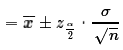.

Please note that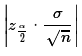is called the margin of error. The absolute value sign is there just so that we always get a positive value for the margin of error. The width of the confidence interval is always two times the margin of error.

We really have two ways that we can change the size of the margin of error and, thus, change the width of the confidence interval. The first is by changing the size of the sample. The larger we make the sample size, n, the larger is the denominator in the margin of error. The larger the denominator, the smaller the margin of error. Back in Figure 3 we never expressed the margin of error as a separate value. In Figure 4 we find and display that value. Then we recompute samp_sd using a sample size of 60. After that we can recompute and display a new margin of error based on that new sample size.

Figure 4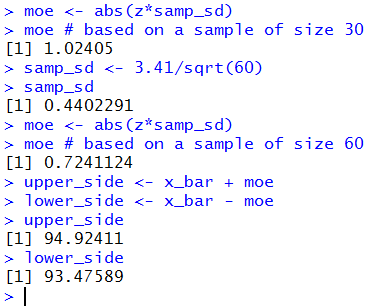By increasing the sample size from 30 to 60 we changed the standard deviation of the sample means from 0.622578 (in Figure 3) to 0.4402291 (in Figure 4). This changes the margin of error from 1.02405 to 0.7241124. Clearly, we can make the margin of error as small as we want by increasing the sample size. But more on that later.

The other way that we can change the margin of error is to change the desired confidence level. Figure 3 starts with us finding the required z-score so that we have 90% of the area in the interval. If we were to change that so that we only wanted 80% of the area in the interval then the value of |z| will be smaller, that is, the z-score will be closer to 0. This, in turn would make the margin of error smaller. Figure 5 redoes the computations in our problem, still using sample size 60, but now with a confidence level set to 80%.

Figure 5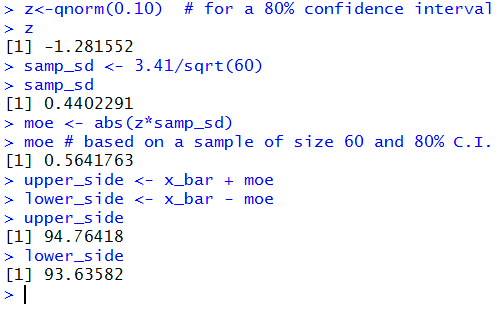Clearly, reducing the sample size or raising the confidence level will widen the confidence interval.

#### Look at some examples

In a previous web page we were able to look at a population of 1000 values where we set the population shape, mean, and standard deviation. And we were able to draw 1000 random samples of some specified size and see the sample mean (and sample standard deviation) for each of those 1000 samples. We will build on that by taking each of those sample means and building a confidence interval from each one with all the confidence intervals being built with the same confidence level.

To do this we need to start at the set up the arguments. page. Figure 6 shows an image of that page with the default values still in place.

Figure 6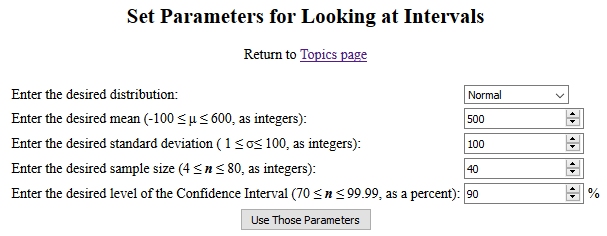For our example we will just use those default values and click on the "Use Those Parameters" button to open a new page with our randomly generated population, our 1000 samples, and our 1000 confidence intervals, one from each sample mean.

As was true for the previous page, all of this will be too long to display here, but I have saved a pdf version of the page I generated so that you can see the entire page if you want. Also as before, we will "walk through" the page that was generated to point out the various parts. Figures 7 through 13 cover the portion of the page that has a structure identical to that of the earlier page. The new material dealing with confidence intervals starts in Figure 14.

The page starts, as shown in Figure 7, with a confirmation of the requested parameters followed by the first listing of the population data.

Figure 7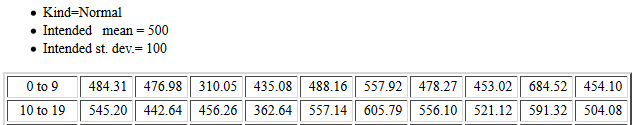At the end of the listing the page displays the mean and standard deviation for those 1000 values in the population. After that is a sorted listing of the original values.

Figure 8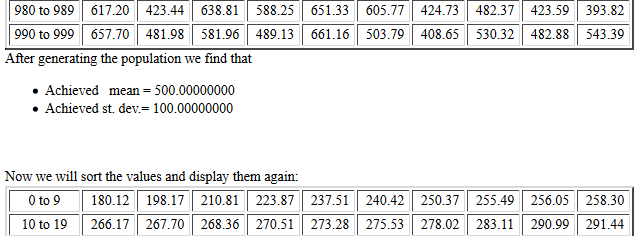Following the sorted listing, the page holds a histogram of the population values, followed by a table giving the boundaries and actual frequencies for each of the rectangles in the histogram. The histogram shown in Figure 9 fits the request for a normal distribution.

Figure 9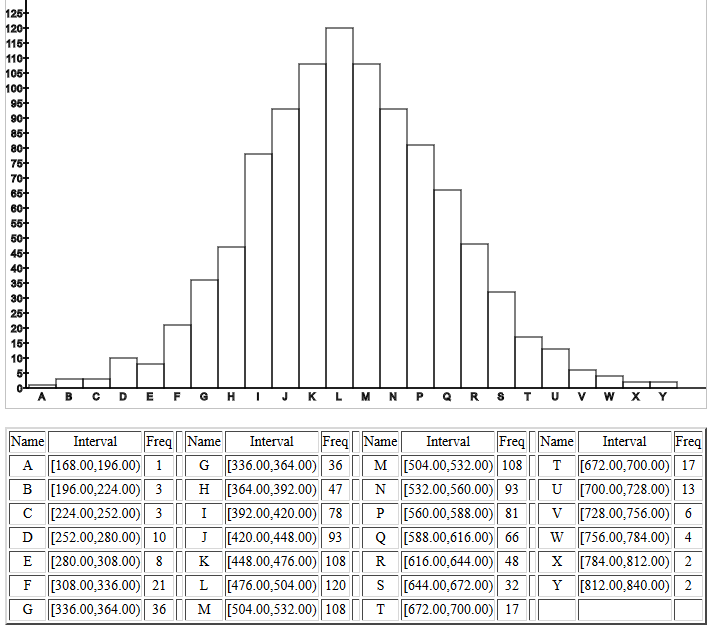There is a "line printer" histogram (not shown here) before the page gets to the start of reporting the mean and standard deviation of the 1000 samples, each of the requested size 40. We are most interested in the sample means. Figure 10 shows the first 40 such sample means.

Figure 10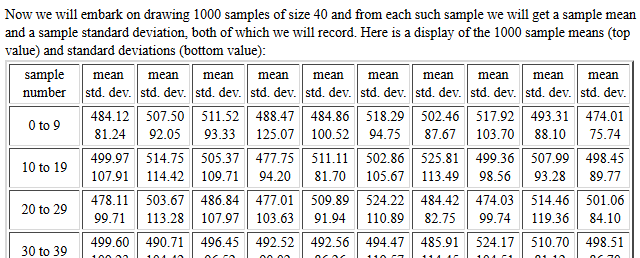The listing of the sample statistic is followed by the information shown in Figure 11. In this case we see that the mean of the 1000 sample means is, as expected, essentially the same as the population mean. Furthermore, the standard deviation of the sample means is about 15.96755, a value quite close to the value predicted by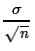, 15.81138830.

Figure 11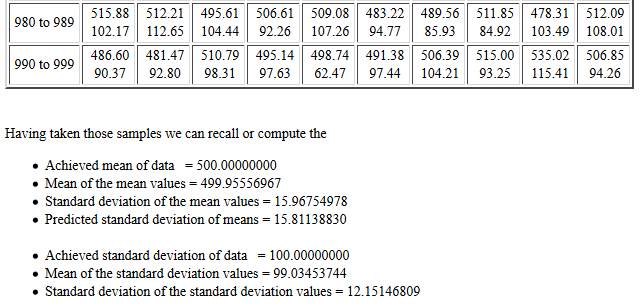This is followed by a histogram of the sample means, reproduced in Figure 12.

Figure 12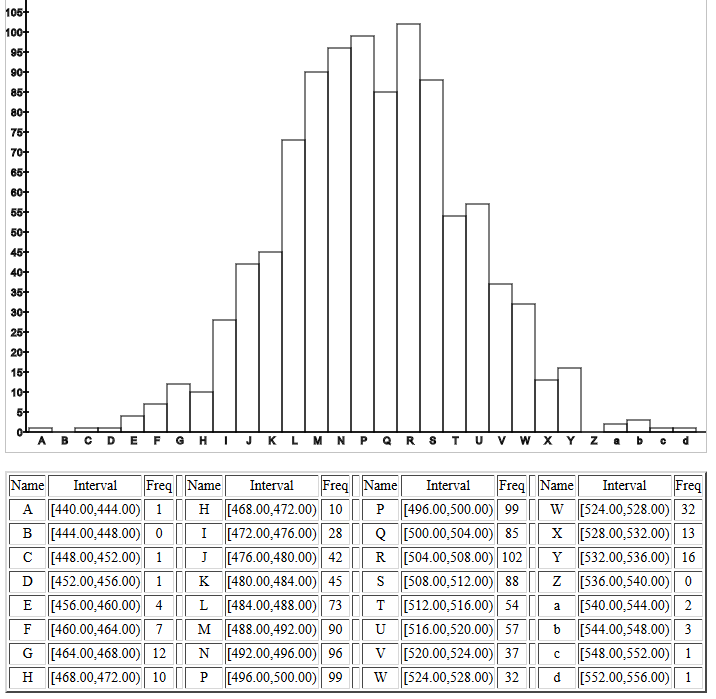Following that histogram is a "line printer" version (again not shown here) after which is a quick report, Figure 13, of the lowest and highest of the 1000 sample means. Up to this point, this page has the identical structure to that of the page that we used to demonstrate taking samples.

Figure 13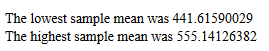Important Notice: This page was designed to show more than the material presented thus far. In particular, we are looking at the confidence intervals generated when the standard deviation of the population is known. Therefore, we will not be discussing the meaning of and the results for confidence intervals when the population standard deviation is unknown. That will be done in a subsequent page.
Figure 14 holds the introduction to the next part of the page.

Figure 14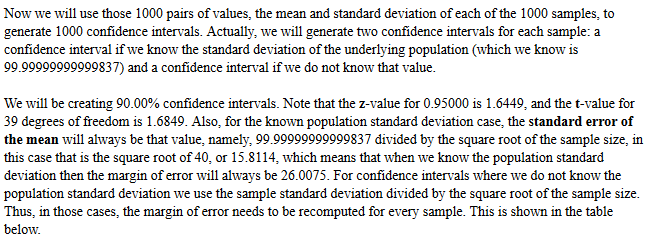Part of that introduction is an explanation to show that for all of the confidence intervals of interest here, the margin of error will be the same, namely 26.0075. Figure 15 shows the first few lines of the table of confidence intervals, one for each of the samples taken. As noted above, here we are only concerned with the left portion of the table, the highlighted portion in Figure 15.

Figure 15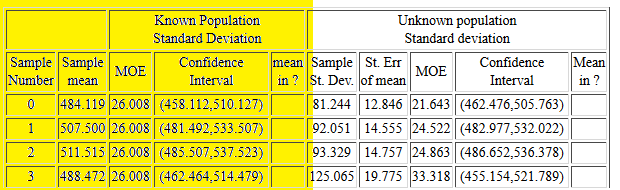Examining Sample Number 0 in Figure 15 we see that the mean of that sample was 484.119, the value first reported back in Figure 10, though there it was given with only 2 decimal places. The margin of error is reported as 26.008. Then the confidence interval is (458.112,510.127). The next column is left blank because the true population mean, 500, is in this confidence interval.

Sample number 1 has a mean of 507.500 which produces confidence interval of (481.492,533.507), which also includes the population mean, 500. Remember that the page generated 90% confidence intervals. Therefore, about 90% of the intervals generated should contain the mean of the population. All 4 of the samples reported in Figure 15 generate confidence intervals that contain the population mean.

Taking samples is a random event. As it turned out in this case, the first time we get a sample that does not contain the true mean is sample case number 40. That is not shown here, but you can see it in the pdf file. Instead, in Figure 16, we can see the second and third instances, samples #65 and #69, where the generated confidence interval does not contain the true mean. The fifth column in each of those is marked FAIL. If you look at the pdf file and scan through all 1000 generated confidence intervals it is easy to pick out the ones that have FAIL to indicate that they did not contain the true population mean.

Figure 16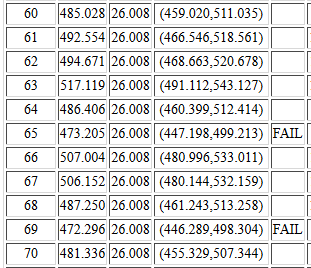What happened in cases #65 and #69 is that the randomly selected 40 values that made up the sample produced a sample mean that was so low that the generated confidence interval, which has a margin of error = 26.008, does not contain the value 500, the mean of the population. There are other cases (#75, #106, #110, ...) where the sample mean is too high and again the confidence interval misses the true mean.

At the end of the table of confidence intervals the web page ends with a count of the number of intervals that failed to include the true mean. This example page was constructed to get 90% confidence intervals. Therefore, we expect that about 90% of the intervals generated will contain the true mean. That leaves 10% where the interval would not contain the true mean. We took 1000 samples. 10% of 1000 is 100. We expect about 100 of our intervals to miss the true mean. Figure 17 reports that indeed 94 of our intervals missed the true mean.

Figure 17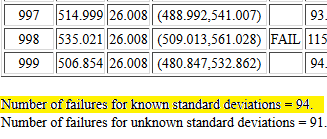It is worth the time and effort to go back and use those web pages to generate numerous populations and samples and confidence intervals, at different confidence levels, and to see the results which will be like those we have just seen in our walk through in Figures 6 through 17.

#### Automating the process

The process of computing a confidence interval in the case where we know the population standard deviation and where we have a sample of size n that yields a sample meanis as follows:
1. From the confidence level compute the value ofusing2. Use qnorm() to find the associated z-score,3. Find the margin of error as4. Find the two parts to the confidence interval by evaluatingWe should be able to describe these same actions using R statements inside an R function. Consider the following function definition:
```ci_known <- function( sigma=1, n=30, x_bar=0, cl=0.95)
{
# try to avoid some common errors
if( cl <=0 | cl>=1)
{return("Confidence interval must be strictly between 0.0 and 1")
}
if( sigma < 0 )
{return("Population standard deviation must be positive")}
if( n <= 1 )
{return("Sample size needs to be more than 1")}
if( as.integer(n) != n )
{return("Sample size must be a whole number")}
# to get here we have some "reasonable" values
samp_sd <- sigma/sqrt(n)
z <- abs( qnorm( (1-cl)/2))
moe <- z*samp_sd
low_end <- x_bar - moe
high_end <- x_bar + moe
result <- c(low_end, high_end, moe, samp_sd)
names(result)<-c("CI Low","CI High","MOE", "Std Error")
return( result )
}
```
This does all of our tasks, including returning the confidence interval as well as some other values. The function is available in the file ci_known.R. Figure 18 shows the console screen after the function has been entered into an R session.

Figure 18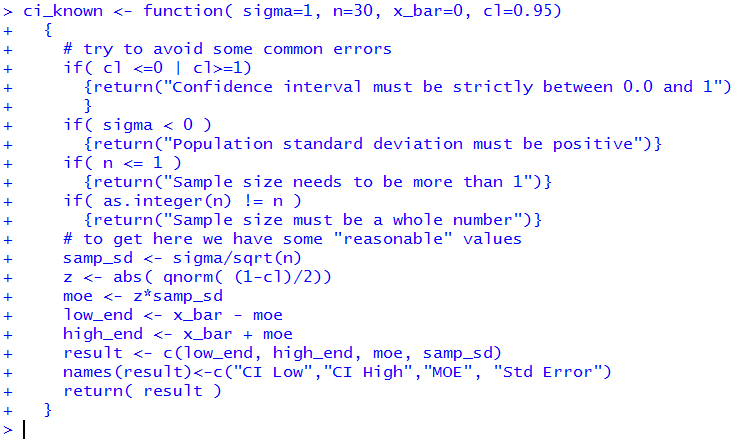Figure 19 shows a use of the ci_known() function to do the problem that we did back in Figure 3. Fortunately, we get the same results.

Figure 19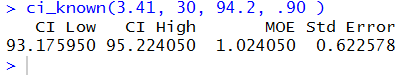Figure 20 uses the information needed to find the 90% confidence intervals for samples #65 and #66 in Figure 16. Again, the function produces the same values that had been on that web page.

Figure 20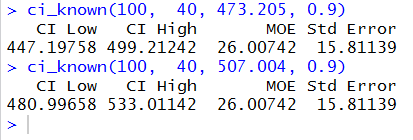If you read the text of the function you know that it tries to trap any obvious errors. Figure 21 shows a number of commands designed to test out those traps. It is interesting to note that the final example, specifying the sample size as 4.0, did not cause an error. Apparently, R has no problem if we use a decimal point in a whole number as long as the value of the number is still an integer.

Figure 21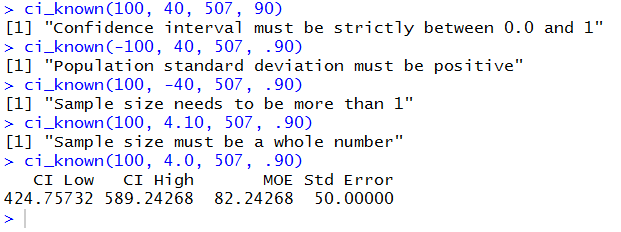It can be difficult to remember the correct order of the arguments in a call to the function. Figure 22 illustrates that we can name the arguments, and, if we do so, we do not have to give them in order. Again, this is the problem first done in Figure 3.

Figure 22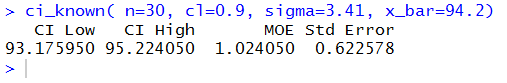The working examples above show the function used by itself. We could assign the results of the function to a variable and then just examine parts or all of that variable. This is done in Figure 23.

Figure 23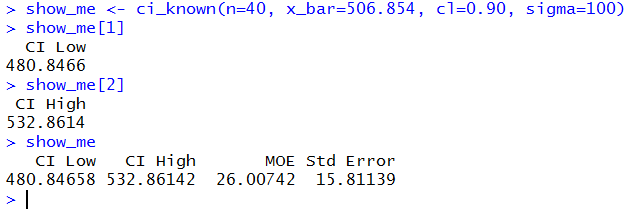And, if we start typing the function (after it has been defined, of course) into an R session then R provides a little note to remind us of the needed arguments. This also shows the default value of each argument. It is true that if we wanted to take the default value then we need not specify it, but that practice should be discouraged.

Figure 24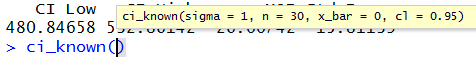#### Interpreting A Confidence Interval

Let us say that we have a population where we know the population standard deviation. We choose a confidence level, perhaps 90%. We take a sample and find the sample mean. From all of that we can compute a 90% confidence interval. It would be wrong to say "There is a 90% chance that the true population mean is within the confidence interval." The true population mean is either in the confidence interval or it is not! It is not a question of probability! What we can and should say is "If I follow the same procedure time and again, then 90% of the confidence intervals that I generate will contain the true mean." That says nothing about the one confidence interval that we did compute. It says a lot about the method of computing it.

#### Worksheet for a Confidence Interval

Here is a link to a worksheet with randomly generated problems related to finding confidence intervals when the standard deviation of the population is known.

```qnorm(0.025)
z<-qnorm(0.05)
z
x_bar <- 94.2
samp_sd <- 3.41/sqrt(30)
samp_sd
lower_side <- x_bar + z*samp_sd
lower_side
upper_side <- x_bar - z*samp_sd
upper_side

moe <- abs(z*samp_sd)
moe # based on a sample of size 30
samp_sd <- 3.41/sqrt(60)
samp_sd
moe <- abs(z*samp_sd)
moe # based on a sample of size 60
upper_side <- x_bar + moe
lower_side <- x_bar - moe
upper_side
lower_side

z<-qnorm(0.10)  # for a 80% confidence interval
z
samp_sd <- 3.41/sqrt(60)
samp_sd
moe <- abs(z*samp_sd)
moe # based on a sample of size 60 and 80% C.I.
upper_side <- x_bar + moe
lower_side <- x_bar - moe
upper_side
lower_side

ci_known <- function( sigma=1, n=30, x_bar=0, cl=0.95)
{
# try to avoid some common errors
if( cl <=0 | cl>=1)
{return("Confidence interval must be strictly between 0.0 and 1")
}
if( sigma < 0 )
{return("Population standard deviation must be positive")}
if( n <= 1 )
{return("Sample size needs to be more than 1")}
if( as.integer(n) != n )
{return("Sample size must be a whole number")}
# to get here we have some "reasonable" values
samp_sd <- sigma/sqrt(n)
z <- abs( qnorm( (1-cl)/2))
moe <- z*samp_sd
low_end <- x_bar - moe
high_end <- x_bar + moe
result <- c(low_end, high_end, moe, samp_sd)
names(result)<-c("CI Low","CI High","MOE", "Std Error")
return( result )
}

ci_known(3.41, 30, 94.2, .90 )
ci_known(100,  40, 473.205, 0.9)
ci_known(100,  40, 507.004, 0.9)
ci_known(100, 40, 507, 90)
ci_known(-100, 40, 507, .90)
ci_known(100, -40, 507, .90)
ci_known(100, 4.10, 507, .90)
ci_known(100, 4.0, 507, .90)

ci_known( n=30, cl=0.9, sigma=3.41, x_bar=94.2)
show_me <- ci_known(n=40, x_bar=506.854, cl=0.90, sigma=100)
show_me
show_me
show_me
```